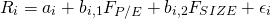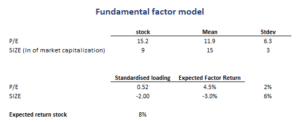## Fundamental Factor Model

A fundamental factor model is a model that is used by analysts to forecast the returns of securities using the fundamentals of the stock. Although fundamental factor models look very similar to macroeconomic factor models, we should stress that the estimation of the models is fundamentally different. In particular, while the macroeconomic model will estimate the sensitivities of stocks to factors, a fundamental factor model calculates the sensitivities from the fundamentals of securities.

While this distinction may sound confusing, we promise that it will be crystal-clear by the end of the article. A numerical example of a fundamental factor model is available at the bottom of the page.

## Fundamental factor model example

Let’s consider a simple fundamental model that we can use to explain the necessary conceptsThe above specification is a two-factor model where we model the stock’s return based on two fundamentals, the P/E ratio and the market capitalization (SIZE) of the company. Now, in the case of a fundamental model, we collect the P/E and SIZE of many companies. These data are then standardized before they are used. These data are the betas (b) or factor loadings. These values measure the “exposure” of a stock to the factors.

The factor returns (the F parameters) in the model are estimated using cross-sectional regressions. These are the factor returns. These returns are equivalent to the returns on a factor that has a unit exposure to the factor, and a zero exposure to the other factors in the model. Thus, the factor’s return is orthogonal to the other factors. The return is also referred to as the return on a factor mimicking portfolio.

This approach is quite different from the approach used to estimate macroeconomic models discussed here. In the case of the macroeconomic models, we estimate the betas (s) using values on F. here, we do the opposite, we estimate F.

Another point we should note is that the intercept no longer has an interpretation in the case of a fundamental model. It is not an expected return (as it is the case in a macroeconomic model).

## Fundamental factor mode example

Finally, let’s apply a factor model. The following table reports the estimation results and loadings on a particular stocks. Using this information, we can calculate the expected return.## Summary

We discussed fundamental factor models. These are widely used by analysts to calculate expected returns. All that we need is a stock’s fundamentals and historical return data.

### Fundamental model

Want to have an implementation in Excel? Download the Excel file: Fundamental model